### Sample Problem

Identify this conic:.

ellipse

parabola

hyperbola

Sketch the graph of this conic.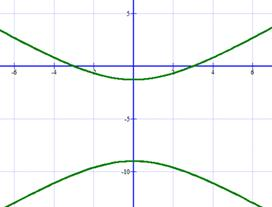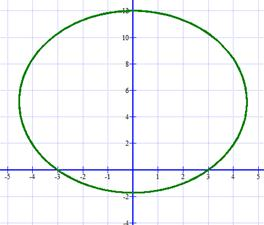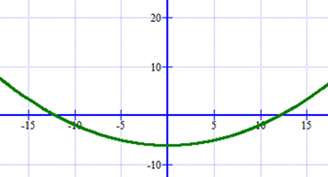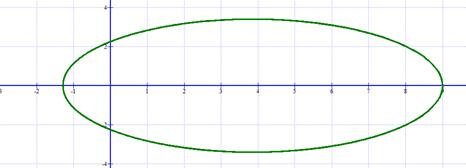#### Solution

To identify the type of conic, we’ll rewrite the equation in the form r=(ep)/(1±ecosθ)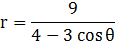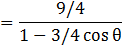e=3/4<1, therefore we know that this graph is an ellipse.

As for the graph of this ellipse, we know that after plotting a few points from θ=0 to θ=π, the ellipse has a horizontal major axis and vertices that lie at (9, 0) and (9/7, π). So, the length of the major axis is 2a=9+9/7=72/7 → 36/7. To find the length of the minor axis, we can use the equations e=c/a and b2=a2-c2 to conclude

e=c/a → ae=c

b2=a2-c2=a2-(ae)2

b2=a2(1-e2)=(36/7)2(1-(3/4)2)=81/7

b= 9/√7=half of the minor axis – 2b=18/√7

Therefore, our graph should look like